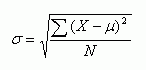Email us to get an instant 20% discount on highly effective K-12 Math & English kwizNET Programs!

#### Online Quiz (WorksheetABCD)

Questions Per Quiz = 2 4 6 8 10

### Middle/High School Algebra, Geometry, and Statistics (AGS)9.27 Standard Deviation - Ungrouped Data

• The standard deviation measures the spread of the data about the mean value.
• It is useful in comparing sets of data which may have the same mean but a different range.
• For example, the mean of the following two is the same: 15, 15, 15, 14, 16 and 2, 7, 14, 22, 30. However, the second is clearly more spread out.
• If a set has a low standard deviation, the values are not spread out too much.
• Just like when working out the mean, the method is different if the data is given to you in groups.
• Non-Grouped Data Non-grouped data is just a list of values. The standard deviation is given by the formula:s means 'standard deviation'
m is the mean
N is the number of values

• Example
Find the standard deviation of 4, 9, 11, 12, 17, 5, 8, 12, 14
The mean of the data is 10.222
Subtracting the mean individually from each of the numbers given and square the result. This is equivalent to thestep. x refers to the values given in the question.
 x 4 9 11 12 17 5 8 12 1438.7 1.49 0.6 3.16 45.9 27.3 4.94 3.16 14.3

Adding up these results = 139.55
Divide by N. is the number of values, so in this case is 9. This gives us: 15.51
And finally, square root this: 3.94
• If you have a set of numbers (e.g. 1, 5, 2, 7, 3, 5 and 3), if each number is increased by the same amount (e.g. to 3, 7, 4, 9, 5, 7 and 5), the standard deviation will be the same and the mean will have increased by the amount each of the numbers were increased by (2 in this case). This is because the standard deviation measures the spread of the data. Increasing each of the numbers by 2 does not make the numbers any more spread out, it just shifts them all along.
Application: In finance, standard deviation is applied to the annual rate of return of an investment to measure the investment's volatility. Standard deviation is also known as historical volatility and is used by investors as a gauge for the amount of expected volatility.

Directions: Answer the following questions. Round the answers to 4 digits after the decimal point. Also write a set of data of your own and find the standard deviation.
 Q 1: Find the standard deviation of 12 and 14Answer: Q 2: Find the standard deviation of 22 and 24Answer: Q 3: Find the standard deviation of 1,2,3Answer: Q 4: Find the standard deviation for the set of numbers: 20, 23, 25, 26. Answer: Question 5: This question is available to subscribers only! Question 6: This question is available to subscribers only!